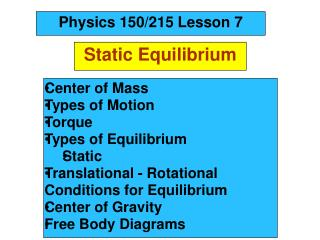DownloadDownload PresentationStatic Equilibrium

# Static Equilibrium

Télécharger la présentation## Static Equilibrium

- - - - - - - - - - - - - - - - - - - - - - - - - - - E N D - - - - - - - - - - - - - - - - - - - - - - - - - - -
##### Presentation Transcript

1. Physics 150/215 Lesson 7 Static Equilibrium • Center of Mass • Types of Motion • Torque • Types of Equilibrium • Static • Translational - Rotational Conditions for Equilibrium • Center of Gravity • Free Body Diagrams

2. Center of Mass = Average position of the masses For example: 6 kg at x=5m & 7 kg at x=10m x coordinate of center of mass = Think of the average height of 6 monsters that are 5m high and 7 that are 10 m high

3. Motion of an object can be analyzed into three types • translation of the center of mass • rotation (rigid) about the center of mass • vibration (elastic) about center of mass

4. Translational Acceleration is caused by the total force on the object If the total force FTot= 0 then there is no Translational Acceleration (acceleration of center of mass) acm = 0

5. Thus vcm = constant • If velocity of center of mass is initially zero it will stay zero • There may be rotation or vibrational motion around center of mass

6. Rotational acceleration is caused by the total turning force = TORQUE about the center of mass.

8. Linear = Translational • Angular = Rotational Total Force = 0; Total Torque not zero Total Force not 0; Total Torque = 0

9. If the total torque Tot= 0 then there is no Rotational Acceleration (change of angular speed) angular acceleration = 0

10. Thus angular speed = constant • If angular speed is initially zero • it will stay zero

11. If object is RIGID There will be no vibration about center of mass

12. Static Equilibrium for rigid objects vcm = 0  = 0 FTot = 0 Tot = 0

13. Conditions for Static Equilibrium for Rigid Objects

14. The center of mass of an object will not accelerate if the total force on the object is zero if Ftot   a  0  TRANSLATIONAL EQUILIBRIUM cm An object will have zero angular acceleration if the total torque on the object is zero if     0  ROTATIONAL EQUILIBRIUM

15. If the initial velocity of the center of mass is zero and the initial angular velocity is zero they will remain zero if Ftot  and acm  0 When this is so the object is said to be in STATIC EQUILIBRIUM

16. Properties of Torque

17. The torque of a force about any point on . the line of action of that force is zero If a body is in translational equilibrium and the net torque is zero with respect to one point in the body then it is zero with respect to all points in the body . forces

18. Center of Gravity The position where the force of gravity acts on the object or rigidly connected objects

19. Free Body Diagrams F1 N1 N2 W1 W2 F2